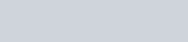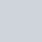The probability of throwing a number greater than 2 with a fair dice is
Question:

The probability of throwing a number greater than 2 with a fair dice is

(a) $\frac{3}{5}$

(b) $\frac{2}{5}$

(c) $\frac{2}{3}$

(d) $\frac{1}{3}$

Solution:

GIVEN: A dice is thrown once

TO FIND: Probability of getting a number greater than 2.

Total number on a dice is 6.

Number greater than 2 is 3, 4, 5 and 6

Total number greater than 2 is 4

We know that PROBABILITY =Hence probability of getting a number greater than 2 is equal toHence the correct option is